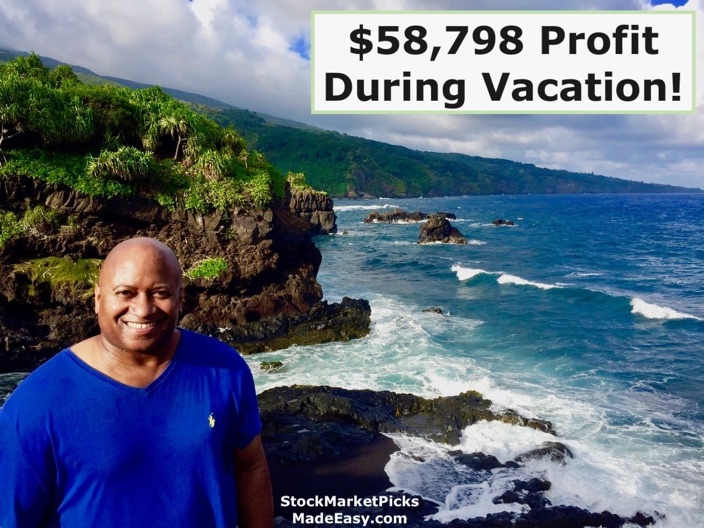Aloha! See how I earned \$58,798 profit on the easy trades below while on vacation!After some quick training, you will start seeing just as many winners as shown on my impressive list here.

Total profit: \$58,798

1. RHE = \$1,986 profit

2. MDB 1 = \$3,100 profit

3. MDB 2 = \$1,540 profit

4. MDB 3 = \$1,770 profit

5. CRM 1 = \$1,820 profit

6. CRM 2 = \$3,628 profit

7. CRM 3 = \$1,574 profit

8. CRM 4 = \$2,252 profit

9. LULU 1 = \$1,320 profit

10. LULU 2 = \$5,590 profit

11. LULU 3 = \$4,515 profit

12. LULU 4 = \$4,635 profit

13. AMD 1 = \$3,700 profit

14. AMD 2 = \$5,664 profit

15. AMD 3 = \$6,564 profit

16. NOW = \$2,550 profit

17. SPLK = \$2,500 profit

18. ROKU = \$1,510 profit

19. TGT = \$2,580 profit